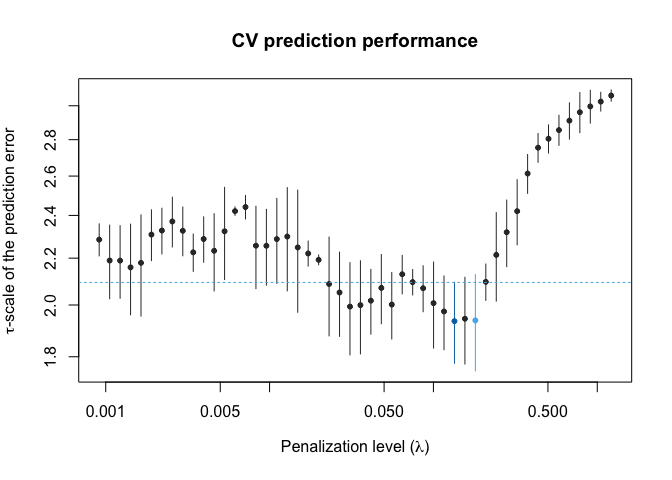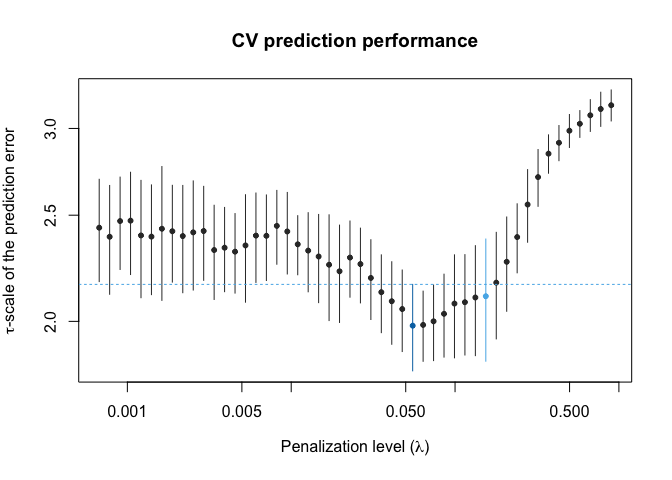# Estimating predictive models

#### 2020-10-03

In this guide we will estimate predictive models by means of robust adaptive PENSE estimates for high-dimensional linear regression. These estimates can tolerate up to 50% of contamination, i.e., the adaptive PENSE estimates are reliable even if up to half the observations in the data set contain anomalous values. Compute adaptive PENSE estimates is implemented in the function adapense_cv() in the pense package.

While the following guide computes adaptive PENSE estimates, everything also applies to other estimates implemented in the pense package: non-adaptive PENSE estimates and regularized M-estimates. Non-adaptive PENSE estimates (computed by pense_cv()) are typically better at identifying all relevant predictors than adaptive PENSE. However, this comes at the price of often including a large number of irrelevant predictors as well. Regularized M-estimates (computed by pensem_cv()) can be more accurate than either PENSE or adaptive PENSE estimates, but may be unreliable in presence of highly detrimental contamination.

First we need to load the pense package:

library(pense)
#> Loading required package: Matrix

Computing robust, regularized estimates for high-dimensional linear regression models can take a long time. Luckily many steps can be done in parallel to save time. If your computer has more than 1 CPU core, you can harness more computing power by creating a cluster of R processes:

library(parallel)
# If you don't know how many CPU cores are available, first run detectCores(logical = FALSE)
cluster <- makeCluster(3)

This guide uses the following simulated data with 50 observations and 40 available predictors. The error distribution is a heavy-tailed t-distribution and only the first 3 predictors are truly relevant for predicting the response y:

set.seed(1234)
x <- matrix(rweibull(50 * 40, 2, 3), ncol = 40)
y <- 1 * x[, 1] - 1.1 * x[, 2] + 1.2 * x[, 3] + rt(nrow(x), df = 2)

To make the scenario more realistic, let’s add some contamination to the response value of the first 3 observations and to some predictors:

y[1:3] <- 5 * apply(x[1:3, ], 1, max)
x[3:6, 4:6] <- 1.5 * max(x) + abs(rcauchy(4 * 3))

## Step 1: Computing the estimates

The first step is to compute adaptive PENSE estimates for a fixed value for hyper-parameter alpha and many different penalization levels (hyper-parameter lambda). The adapense_cv() function automatically determines a grid of penalization levels, with parameter nlambda= controlling the number of different penalization levels to be used (default 50). We are going to choose the penalization level which leads to a good balance between prediction accuracy and model size. The pense package can automatically evaluate prediction accuracy of the adaptive PENSE estimates via cross-validation.

In its simplest form, computing adaptive PENSE estimates and estimating their prediction accuracy is done with the code below. Here, prediction accuracy is estimated via 5-fold cross-validation, replicated 3 times:

set.seed(1234)
fit_075 <- adapense_cv(x, y, alpha = 0.75, cv_k = 5, cv_repl = 3, cl = cluster)

You should always set a seed prior to computing adaptive PENSE or PENSE estimates to ensure reproducibility of the internal cross-validation.

By default, adaptive PENSE estimates are computed with a breakdown point of 25%. This means, the estimates are reliable if up to 25% of observations contain arbitrary contamination. If you suspect that a larger proportion of observations may be affected by contamination, you can increase the breakdown point of the estimates with argument bdp= to up to 50%. Note, however, that a higher breakdown point also leads to less accurate estimates.

## Step 2: Assessing prediction performance

The plot() function for the object fit_075 shows the estimated prediction accuracy for all fitted models.

plot(fit_075)Estimated prediction accuracy using 3 replications of 5-fold CV.

The plot shows the estimated scale of the prediction error for all 50 models. The penalization level leading to the best prediction performance is highlighted by a dark blue dot. If more than one CV replication was performed, the plot also shows a light blue dot, marking the most parsimonious model with prediction performance “indistinguishable” from the best model. The plot uses the “one-standard-error” rule, visualized by the dashed horizontal line depicting the the minimum average scale of the prediction error plus 1 standard error of this estimated scale. You can adjust this rule to the “q-standard-error” with plot(se_mult = q), where q is any positive number.

In our case, the estimated prediction performance is fairly unstable and has large variability. With such unstable estimates of prediction performance it is difficult to reliably select the penalization level. This is not unusual for robust estimators and can be improved by increasing the number of CV replications.

The more CV replications, the more accurate the estimates of prediction accuracy, but the longer the computing time. If we repeat step #1, but with 10 CV replications instead of 3, we get a more stable evaluation of prediction performance:

set.seed(1234)
fit_075 <- adapense_cv(x, y, alpha = 0.75, cv_k = 5, cv_repl = 10, cl = cluster)
plot(fit_075)Estimated prediction accuracy using 10 replications of 5-fold CV.

With 10 replications, the error bars are still large, a testament of the contamination in the sample, but the general trend of the prediction performance is much clearer. Of course, you could increase the number of CV replications further to get an even smoother plot.

## Step 3: Extracting coefficients

Once we are happy with the stability of the estimated prediction performance, we can extract summary information from the predictive model with

summary(fit_075)
#> Adaptive PENSE fit with prediction performance estimated by 10 replications of
#> 5-fold cross-validation.
#>
#> 14 out of 40 predictors have non-zero coefficients:
#>
#>                 Estimate
#> (Intercept)  1.527069371
#> X1           0.722224241
#> X2          -0.868422718
#> X3           0.794967863
#> X5           0.061834699
#> X12          0.100211936
#> X19         -0.225896936
#> X21          0.304081225
#> X22          0.063632685
#> X26         -0.402350971
#> X29          0.201989891
#> X32         -0.001817195
#> X36         -0.273561877
#> X39         -0.134544249
#> X40         -0.001827169
#> ---
#>
#> Hyper-parameters: lambda=0.05512509, alpha=0.75, exponent=1
#> Estimated scale of the prediction error: 1.982047

This model corresponds to the model with smallest scale of the prediction error (the blue dot in the plot above). There are a total of 14 predictors in the model. If you think a sparser model may be more appropriate for your application, you can also apply the q-standard-error rule as in the plots. The default, the one-standard-error rule leads to the following predictive model:

summary(fit_075, lambda = "se")
#> Adaptive PENSE fit with prediction performance estimated by 10 replications of
#> 5-fold cross-validation.
#>
#> 7 out of 40 predictors have non-zero coefficients:
#>
#>                Estimate
#> (Intercept)  0.22807906
#> X1           0.76580403
#> X2          -0.81598826
#> X3           0.85016587
#> X5           0.02493568
#> X22          0.02658272
#> X29          0.10179342
#> X39         -0.07248717
#> ---
#>
#> Hyper-parameters: lambda=0.1540881, alpha=0.75, exponent=1
#> Estimated scale of the prediction error: 2.10859

In this fit, only 7 out of the 40 predictors are relevant, including the 3 truly relevant predictors. But maybe different values for hyper-parameters alpha and exponent lead to even better prediction?

## Step 4: Exploring different hyper-parameters

The choice for hyper-parameters alpha and exponent (which was kept at its default value of 1) are rather arbitrary. The effects of these two hyper-parameters on the estimates are in general less pronounced than of the penalization level. But you may still want to explore different values for alpha and exponent.

While alpha=0.75 is a good value for many applications, alpha=1 may also be of interest, particularly in applications where correlation between predictors is not an issue. In applications with high correlation between predictors, lower values of alpha (e.g., alpha=0.5) may lead to more stability in variable selection.

The hyper-parameter exponent generally has an effect on the sparsity of the models. With higher values for exponent, typically only predictors with the largest (in an absolute sense) standardized coefficients will be non-zero. While this helps to screen out many or most of the truly irrelevant, it also risks missing some of the truly relevant predictors.

Let us compute adaptive PENSE estimates for different values of hyper-parameters alpha and exponent. In the code below, this is done by manually iterating two different values for our hyper parameters: alpha=0.75 and alpha=1 as well as exponent=1 and exponent=2. For more values of the hyper-parameters, iterating over all the possible combinations would be easier.

set.seed(1234)
fit_075_2 <- adapense_cv(x, y, alpha = 0.75, exponent = 2, cv_k = 5, cv_repl = 10, cl = cluster)

set.seed(1234)
fit_100_1 <- adapense_cv(x, y, alpha = 1, exponent = 1, cv_k = 5, cv_repl = 10, cl = cluster)

set.seed(1234)
fit_100_2 <- adapense_cv(x, y, alpha = 1, exponent = 2, cv_k = 5, cv_repl = 10, cl = cluster)

Note that we set the seed before each call to adapense_cv(). This is again to ensure reproducibility of CV, but also to make the estimated prediction performance more comparable across different values of the hyper-parameters.

After checking that the cross-validated prediction performance of the fitted models is smooth enough to reliably select the penalization level, we can compare all the estimates. For this, the package includes the function prediction_performance(), which extracts and prints the prediction performance of all given objects:

prediction_performance(fit_075, fit_075_2, fit_100_1, fit_100_2)
#> Prediction performance estimated by cross-validation:
#>
#>       Model Estimate Std. Error Predictors alpha exp.
#> 1 fit_075_2 1.864024  0.2022757         14  0.75    2
#> 2 fit_100_2 1.864606  0.2010057         14  1.00    2
#> 3   fit_075 1.982047  0.1795832         14  0.75    1
#> 4 fit_100_1 1.988588  0.1466705         12  1.00    1

Here we see the combination of hyper-parameters alpha=0.75 and exponent=1 (in object fit_075_2) lead to the best prediction accuracy, but the models are all based on more than 12 predictors. Instead, we can also compare sparser models with almost the same prediction performance:

prediction_performance(fit_075, fit_075_2, fit_100_1, fit_100_2, lambda = 'se')
#> Prediction performance estimated by cross-validation:
#>
#>       Model Estimate Std. Error Predictors alpha exp.
#> 1 fit_075_2 2.027702  0.2323029          4  0.75    2
#> 2 fit_100_2 2.045782  0.2314871          4  1.00    2
#> 3 fit_100_1 2.081398  0.2771445          7  1.00    1
#> 4   fit_075 2.108590  0.2692151          7  0.75    1

Still, the combination of hyper-parameters alpha=0.75 and exponent=1 outperforms the others. We can see that the estimated coefficients and estimated relevant predictors are close to the truth:

summary(fit_075_2, lambda = 'se')
#> Adaptive PENSE fit with prediction performance estimated by 10 replications of
#> 5-fold cross-validation.
#>
#> 4 out of 40 predictors have non-zero coefficients:
#>
#>                Estimate
#> (Intercept)  0.42755964
#> X1           0.84993695
#> X2          -0.91559693
#> X3           0.85350171
#> X21          0.02125563
#> ---
#>
#> Hyper-parameters: lambda=0.02962154, alpha=0.75, exponent=2
#> Estimated scale of the prediction error: 2.027702

## Using different measures of prediction performance

By default, adapense_cv() uses the τ-scale of the prediction errors to assess prediction accuracy. This can be changed by specifying a different metric via adapense_cv(cv_metric=). The package supports also the median absolute prediction error (cv_metric = "mape") or the classical root mean squared prediction error (cv_metric = "rmspe"). You should, however, not use the RMSPE to evaluate prediction performance in the potential presence of contamination. Robust methods are not designed to predict contaminated observations well and the RMSPE may be artificially inflated by poor prediction of a few contaminated response values. You can also specify your own function which takes as input the vector of prediction errors and returns a single number, measuring the prediction performance. For example, to use the mean absolute prediction error, you would write

mae <- function (prediction_errors) {
mean(abs(prediction_errors))
}

set.seed(1234)
fit_075_mae <- adapense_cv(x, y, alpha = 0.75, cv_k = 5, cv_repl = 5, cl = cluster, cv_metric = mae)

A matrix with estimates of the prediction performance are accessible as slot \$cv_replications in the object returned by adapense_cv(). The rows correspond to the different penalization levels, and columns correspond to the individual CV replications.